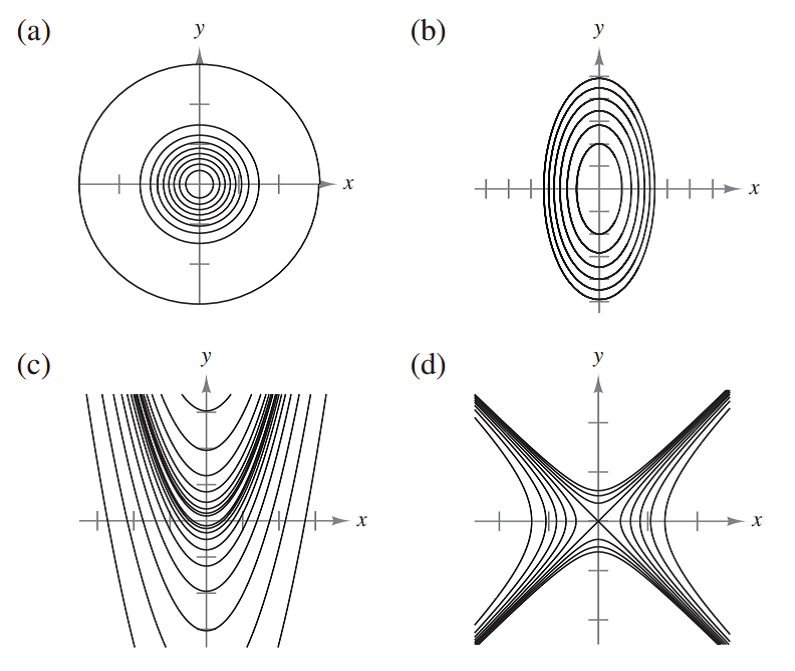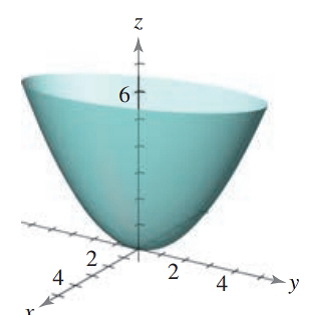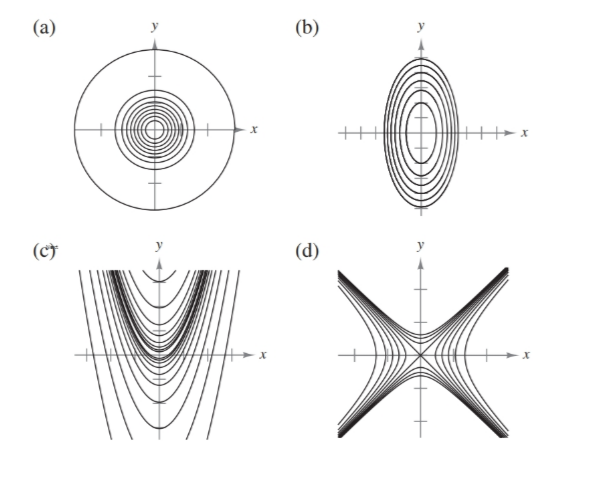Chapter 7.3, Problem 31E### Calculus: An Applied Approach (Min...

10th Edition
Ron Larson
ISBN: 9781305860919

#### Solutions

Chapter
Section### Calculus: An Applied Approach (Min...

10th Edition
Ron Larson
ISBN: 9781305860919
Textbook Problem
1 views

# Matching In Exercises 31-34, match the graph of the surface with one of the contour maps. [The contour maps are labeled (a)-(d).]f ( x , y ) = x 2 + y 2 4To determine

The correct match for the function f(x,y)=x2+y24 from the following options:Explanation

Given Information:

The provide function f(x,y)=x2+y24 is options are:

The graph of equation f(x,y)=x2+y24 given bellows,.

Consider the provide function,

f(x,y)=x2+y24

Substitute f(x,y)=C1 in the equation f(x,y)=x2+y24 to obtain contour map,

C1=x2+y24

This equation represent the equation of ellipse.

Substitute f(x,y)=C1 in the equation f(x,y)=x2+y24 to obtain contour map,

C2=x2+y24

This equation represent the equation of ellipse

### Still sussing out bartleby?

Check out a sample textbook solution.

See a sample solution

#### The Solution to Your Study Problems

Bartleby provides explanations to thousands of textbook problems written by our experts, many with advanced degrees!

Get Started